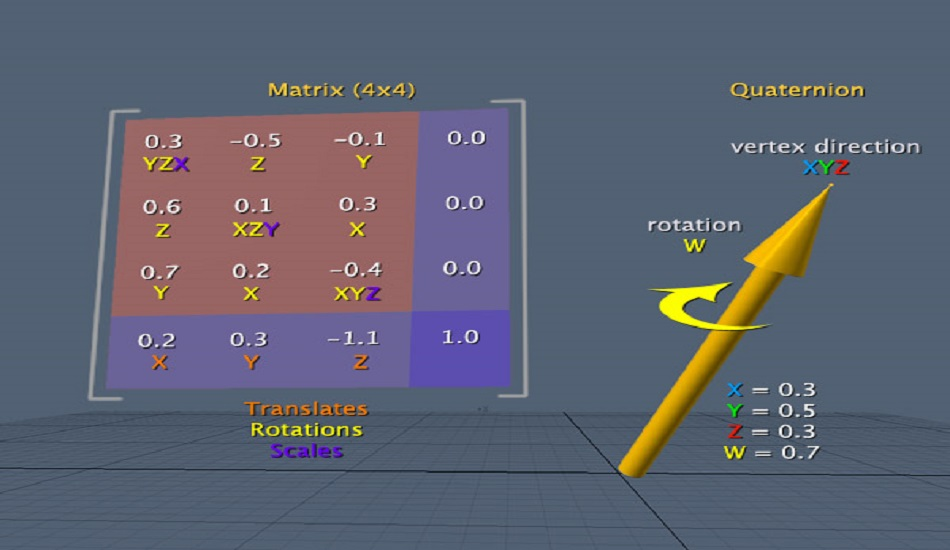# Academics

## Guest Lecture on Matrix Transformation Organized by Department of Mathematics.

Professor Manjul Gupta, Department of Mathematics, I.I.T., Kanpur, delivered a lecture on “Matrix Transformation” on January 25, 2018 at 11:00 a.m. in the Conference Hall-4 (Central Library building).A transformation matrix is a special matrix that can describe 2d and 3d transformations. Transformations are frequently used in linear algebra and computer graphics, since transformations can be easily represented, combined and computed.In linear algebra, linear transformation can be represented by Matrices. If T is a linear transformation mapping Rn to Rm and is a column vector with n entries, then for some m×n matrix A, called the Transformation Matrix of T. There are alternative expressions of transformation matrices involving row vectors that are preferred by some.

Professor Manjul Gupta shall introduce basics of duality of vector spaces, Schauder bases, sequence spaces and then representation of a linear map defined on sequence spaces as an infinite matrix.